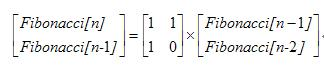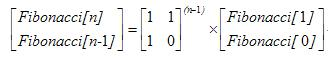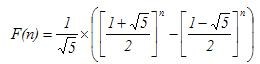# 斐波那契数列算法分析

1，有一段楼梯有10级台阶,规定每一步只能跨一级或两级,要登上第10级台阶有几种不同的走法?

2，数列中相邻两项的前项比后项的极限是多少，就是问，当n趋于无穷大时，F(n)/F(n+1)的极限是多少？

Fibonacci数列的数学表达式就是：

F(n) = F(n-1) + F(n-2)

F(1) = 1

F(2) = 1

Fibonacci数列可以用很直观的二叉递归程序来写，用C++语言的描述如下：

long fib1(int n)

{

if (n <= 2)

{

return 1;

}

else

{

return fib1(n-1) + fib1(n-2);

}

}

long fib1(int n, int* arr)

{

arr[n]++;

if (n <= 2)

{

return 1;

}

else

{

return fib1(n-1, arr) + fib1(n-2, arr);

}

}

fib(10) = 1     fib(9) = 1      fib(8) = 2      fib(7) = 3

fib(6) = 5      fib(5) = 8      fib(4) = 13    fib(3) = 21

fib(2) = 34   fib(1) = 55    fib(0) = 34

T(N) = T(N-1) + T(N-2) + 2

fib(N) < (5/3)^N

long fib(int n, long a, long b, int count)

{

if (count == n)

return b;

return fib(n, b, a+b, ++count);

}

long fib2(int n)

{

return fib(n, 0, 1, 1);

}

Fibonacci数列用迭代程序来写也很容易，用C++语言的描述如下：

//也可以用数组将每次计算的f(n)存储下来，用来下次计算用（空间换时间）

long fib3 (int n)

{

long x = 0, y = 1;

for (int j = 1; j < n; j++)

{

y = x + y;

x = y - x;

}

return y;

}

Fibonacci = 0，Fibonacci = 1

Fibonacci[n] = Fibonacci[n-1] + Fibonacci[n-2] (n >= 2)class Matrix

{

public:

long matr;

Matrix(const Matrix&rhs);

Matrix(long a, long b, long c, long d);

Matrix& operator=(const Matrix&);

friend Matrix operator*(const Matrix& lhs, const Matrix& rhs)

{

Matrix ret(0,0,0,0);

ret.matr = lhs.matr*rhs.matr + lhs.matr*rhs.matr;

ret.matr = lhs.matr*rhs.matr + lhs.matr*rhs.matr;

ret.matr = lhs.matr*rhs.matr + lhs.matr*rhs.matr;

ret.matr = lhs.matr*rhs.matr + lhs.matr*rhs.matr;

return ret;

}

};

Matrix::Matrix(long a, long b, long c, long d)

{

this->matr = a;

this->matr = b;

this->matr = c;

this->matr = d;

}

Matrix::Matrix(const Matrix &rhs)

{

this->matr = rhs.matr;

this->matr = rhs.matr;

this->matr = rhs.matr;

this->matr = rhs.matr;

}

Matrix& Matrix::operator =(const Matrix &rhs)

{

this->matr = rhs.matr;

this->matr = rhs.matr;

this->matr = rhs.matr;

this->matr = rhs.matr;

return *this;

}

Matrix power(const Matrix& m, int n)

{

if (n == 1)

return m;

if (n%2 == 0)

return power(m*m, n/2);

else

return power(m*m, n/2) * m;

}

long fib4 (int n)

{

Matrix matrix0(1, 1, 1, 0);

matrix0 = power(matrix0, n-1);

return matrix0.matr;

}

O1的时间就能求得到F(n)了：long fib5(int n)

{

double z = sqrt(5.0);

double x = (1 + z)/2;

double y = (1 - z)/2;

return (pow(x, n) - pow(y, n))/z + 0.5;

}

int main()

{

cout << fib1(45) << endl;

cout << fib2(45) << endl;

cout << fib3(45) << endl;

cout << fib4(45) << endl;

cout << fib5(45) << endl;

return 0;

}

#include <iostream>

#include <vector>

#include <string>

#include <cmath>

#include <fstream>

using namespace std;

class Matrix

{

public:

long matr;

Matrix(const Matrix&rhs);

Matrix(long a, long b, long c, long d);

Matrix& operator=(const Matrix&);

friend Matrix operator*(const Matrix& lhs, const Matrix& rhs)

{

Matrix ret(0,0,0,0);

ret.matr = lhs.matr*rhs.matr + lhs.matr*rhs.matr;

ret.matr = lhs.matr*rhs.matr + lhs.matr*rhs.matr;

ret.matr = lhs.matr*rhs.matr + lhs.matr*rhs.matr;

ret.matr = lhs.matr*rhs.matr + lhs.matr*rhs.matr;

return ret;

}

};

Matrix::Matrix(long a, long b, long c, long d)

{

this->matr = a;

this->matr = b;

this->matr = c;

this->matr = d;

}

Matrix::Matrix(const Matrix &rhs)

{

this->matr = rhs.matr;

this->matr = rhs.matr;

this->matr = rhs.matr;

this->matr = rhs.matr;

}

Matrix& Matrix::operator =(const Matrix &rhs)

{

this->matr = rhs.matr;

this->matr = rhs.matr;

this->matr = rhs.matr;

this->matr = rhs.matr;

return *this;

}

Matrix power(const Matrix& m, int n)

{

if (n == 1)

return m;

if (n%2 == 0)

return power(m*m, n/2);

else

return power(m*m, n/2) * m;

}

//普通递归

long fib1(int n)

{

if (n <= 2)

{

return 1;

}

else

{

return fib1(n-1) + fib1(n-2);

}

}

/*上面的效率分析代码

long fib1(int n, int* arr)

{

arr[n]++;

if (n <= 1)

{

return 1;

}

else

{

return fib1(n-1, arr) + fib1(n-2, arr);

}

}

*/

long fib(int n, long a, long b, int count)

{

if (count == n)

return b;

return fib(n, b, a+b, ++count);

}

//一叉递归

long fib2(int n)

{

return fib(n, 0, 1, 1);

}

//非递归方法O(n)

long fib3 (int n)

{

long x = 0, y = 1;

for (int j = 1; j < n; j++)

{

y = x + y;

x = y - x;

}

return y;

}

//矩阵乘法O(log(n))

long fib4 (int n)

{

Matrix matrix0(1, 1, 1, 0);

matrix0 = power(matrix0, n-1);

return matrix0.matr;

}

//公式法O(1)

long fib5(int n)

{

double z = sqrt(5.0);

double x = (1 + z)/2;

double y = (1 - z)/2;

return (pow(x, n) - pow(y, n))/z + 0.5;

}

int main()

{

//n = 45时候fib1()很慢

int n = 10;

cout << fib1(n) << endl;

cout << fib2(n) << endl;

cout << fib3(n) << endl;

cout << fib4(n) << endl;

cout << fib5(n) << endl;

return 0;

}